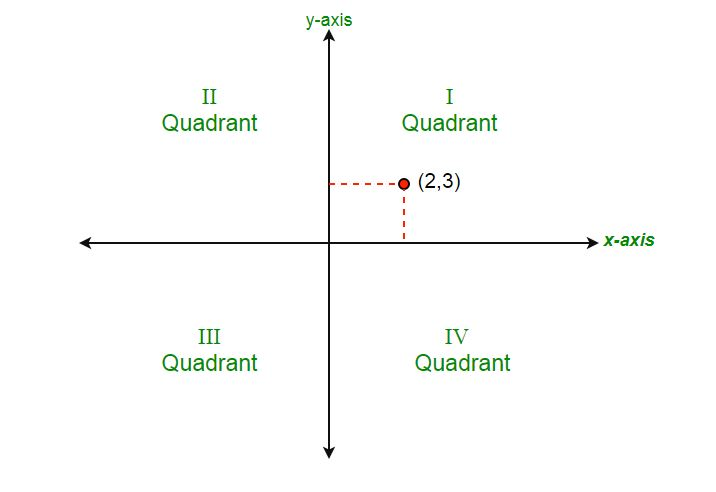# Program to determine the quadrant of the cartesian plane

• Difficulty Level : Basic
• Last Updated : 20 Apr, 2021

Given co-ordinates (x, y), determine the quadrant of the cartesian plane.Image_source : wikipedia.org
Examples :

```Input : x = 1, y = 1
Output : lies in 1st quadrant

Input : x = 0, y = 0
Output : lies at origin```

There are 9 conditions that needs to be checked to determine where does the points lies –

```If in first quadrant then,    x > 0 and y > 0
If in second quadrant then,   x < 0 and y > 0
If in third quadrant then,    x < 0 and y < 0
If in fourth quadrant then,   x > 0 and y < 0
If in positive x-axis then,   y = 0 and x > 0
If in negative x-axis then,   y = 0 and x < 0
If in positive y-axis then,   x = 0 and y > 0
If in negative y-axis then,   x = 0 and y < 0
If at origin then,            x = 0 and y = 0 ```

Below is the implementation of the above approach :

## C++

 `// CPP program to check quadrant``#include ``using` `namespace` `std;` `// Function to check quadrant``void` `quadrant(``int` `x, ``int` `y)``{` `    ``if` `(x > 0 and y > 0)``        ``cout << ``"lies in First quadrant"``;` `    ``else` `if` `(x < 0 and y > 0)``        ``cout << ``"lies in Second quadrant"``;` `    ``else` `if` `(x < 0 and y < 0)``        ``cout << ``"lies in Third quadrant"``;` `    ``else` `if` `(x > 0 and y < 0)``        ``cout << ``"lies in Fourth quadrant"``;` `    ``else` `if` `(x == 0 and y > 0)``        ``cout << ``"lies at positive y axis"``;` `    ``else` `if` `(x == 0 and y < 0)``        ``cout << ``"lies at negative y axis"``;` `    ``else` `if` `(y == 0 and x < 0)``        ``cout << ``"lies at negative x axis"``;` `    ``else` `if` `(y == 0 and x > 0)``        ``cout << ``"lies at positive x axis"``;` `    ``else``        ``cout << ``"lies at origin"``;``}` `// Driver code``int` `main()``{``    ``int` `x = 1, y = 1;``    ``// Function call``    ``quadrant(x, y);``    ``return` `0;``}`

## Java

 `// Java program to check quadrant``import` `java.io.*;` `class` `GFG {``    ` `    ``// Function to check quadrant``    ``static` `void` `quadrant(``int` `x, ``int` `y)``    ``{``        ``if` `(x > ``0` `&& y > ``0``)``            ``System.out.println(``"lies in First quadrant"``);` `        ``else` `if` `(x < ``0` `&& y > ``0``)``            ``System.out.println(``"lies in Second quadrant"``);` `        ``else` `if` `(x < ``0` `&& y < ``0``)``            ``System.out.println(``"lies in Third quadrant"``);` `        ``else` `if` `(x > ``0` `&& y < ``0``)``            ``System.out.println(``"lies in Fourth quadrant"``);` `        ``else` `if` `(x == ``0` `&& y > ``0``)``            ``System.out.println(``"lies at positive y axis"``);` `        ``else` `if` `(x == ``0` `&& y < ``0``)``            ``System.out.println(``"lies at negative y axis"``);` `        ``else` `if` `(y == ``0` `&& x < ``0``)``            ``System.out.println(``"lies at negative x axis"``);` `        ``else` `if` `(y == ``0` `&& x > ``0``)``            ``System.out.println(``"lies at positive x axis"``);` `        ``else``            ``System.out.println(``"lies at origin"``);``    ``}``    ` `    ``// Driver Code``    ``public` `static` `void` `main(String[] args)``    ``{``        ``int` `x = ``1``, y = ``1``;``        ``// Function Calling``        ``quadrant(x, y);``    ``}``}`

## Python

 `''' Python program to check quadrant'''` `# Function to check quadrant``def` `quadrant(x, y):``    ``if` `(x > ``0` `and` `y > ``0``):``        ``print` `(``"lies in First quadrant"``)` `    ``elif` `(x < ``0` `and` `y > ``0``):``        ``print` `(``"lies in Second quadrant"``)``        ` `    ``elif` `(x < ``0` `and` `y < ``0``):``        ``print` `(``"lies in Third quadrant"``)``    ` `    ``elif` `(x > ``0` `and` `y < ``0``):``        ``print` `(``"lies in Fourth quadrant"``)``        ` `    ``elif` `(x ``=``=` `0` `and` `y > ``0``):``        ``print` `(``"lies at positive y axis"``)``    ` `    ``elif` `(x ``=``=` `0` `and` `y < ``0``):``        ``print` `(``"lies at negative y axis"``)``    ` `    ``elif` `(y ``=``=` `0` `and` `x < ``0``):``        ``print` `(``"lies at negative x axis"``)``    ` `    ``elif` `(y ``=``=` `0` `and` `x > ``0``):``        ``print` `(``"lies at positive x axis"``)``    ` `    ``else``:``        ``print` `(``"lies at origin"``)` `# Driver code    ``x ``=` `1``y ``=` `1``quadrant(x, y)`

## C#

 `// C# program to check quadrant``using` `System;` `class` `GFG {` `    ``// Function to check quadrant``    ``static` `void` `quadrant(``int` `x, ``int` `y)``    ``{``        ``if` `(x > 0 && y > 0)``            ``Console.WriteLine(``"lies in First quadrant"``);` `        ``else` `if` `(x < 0 && y > 0)``            ``Console.WriteLine(``"lies in Second quadrant"``);` `        ``else` `if` `(x < 0 && y < 0)``            ``Console.WriteLine(``"lies in Third quadrant"``);` `        ``else` `if` `(x > 0 && y < 0)``            ``Console.WriteLine(``"lies in Fourth quadrant"``);` `        ``else` `if` `(x == 0 && y > 0)``            ``Console.WriteLine(``"lies at positive y axis"``);` `        ``else` `if` `(x == 0 && y < 0)``            ``Console.WriteLine(``"lies at negative y axis"``);` `        ``else` `if` `(y == 0 && x < 0)``            ``Console.WriteLine(``"lies at negative x axis"``);` `        ``else` `if` `(y == 0 && x > 0)``            ``Console.WriteLine(``"lies at positive x axis"``);` `        ``else``            ``Console.WriteLine(``"lies at origin"``);``    ``}` `    ``// Driver Code``    ``public` `static` `void` `Main()``    ``{``        ``int` `x = 1, y = 1;``        ` `        ``// Function Calling``        ``quadrant(x, y);``    ``}``}` `// This code is contributed by vt_m.`

## PHP

 ` 0 ``and` `\$y` `> 0)``        ``echo``(``"lies in First quadrant"``);` `    ``else` `if` `(``\$x` `< 0 ``and` `\$y` `> 0)``        ``echo``(``"lies in Second quadrant"``);` `    ``else` `if` `(``\$x` `< 0 ``and` `\$y` `< 0)``        ``echo``(``"lies in Third quadrant"``);` `    ``else` `if` `(``\$x` `> 0 ``and` `\$y` `< 0)``        ``echo``(``"lies in Fourth quadrant"``);` `    ``else` `if` `(``\$x` `== 0 ``and` `\$y` `> 0)``        ``echo``(``"lies at positive y axis"``);` `    ``else` `if` `(``\$x` `== 0 ``and` `\$y` `< 0)``        ``echo``(``"lies at negative y axis"``);` `    ``else` `if` `(``\$y` `== 0 ``and` `\$x` `< 0)``        ``echo``(``"lies at negative x axis"``);` `    ``else` `if` `(``\$y` `== 0 ``and` `\$x` `> 0)``        ``echo``(``"lies at positive x axis"``);``    ``else``        ``echo``(``"lies at origin"``);``}` `// Driver code``\$x` `= 1; ``\$y` `= 1;` `// Function call``quadrant(``\$x``, ``\$y``);` `// This code is contributed by Ajit.``?>`

## Javascript

 ``

Output :

`lies in First quadrant`

My Personal Notes arrow_drop_up